# Forces Motion Review Describe Acceleration Describe Acceleration A

• Slides: 44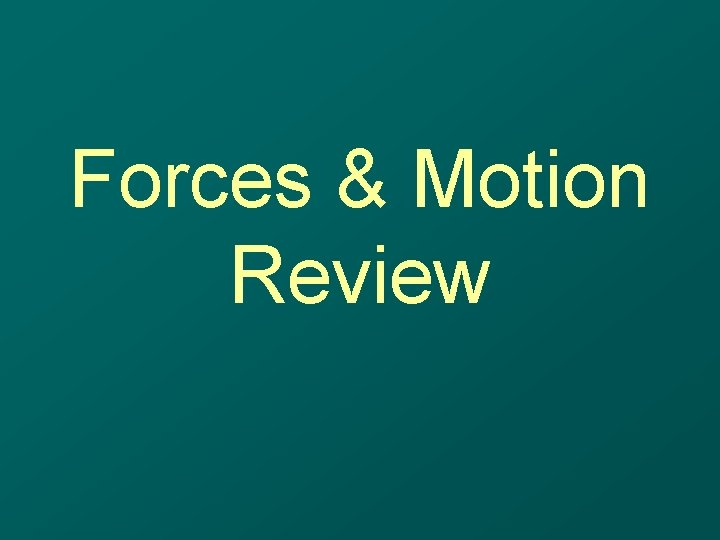Forces & Motion ReviewDescribe AccelerationDescribe Acceleration • A change in velocity – which may be: – A change in speed • • Starting Stopping Speeding up Slowing down – A change in direction • Acceleration is caused by unbalanced forces More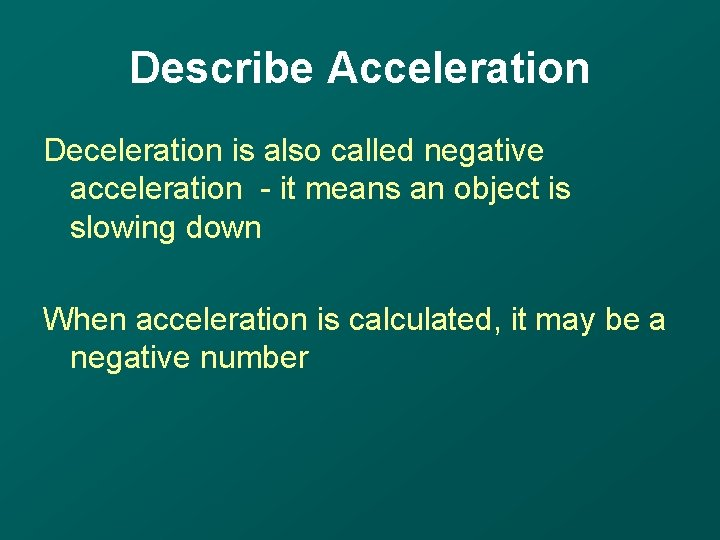Describe Acceleration Deceleration is also called negative acceleration - it means an object is slowing down When acceleration is calculated, it may be a negative numberDescribe Speed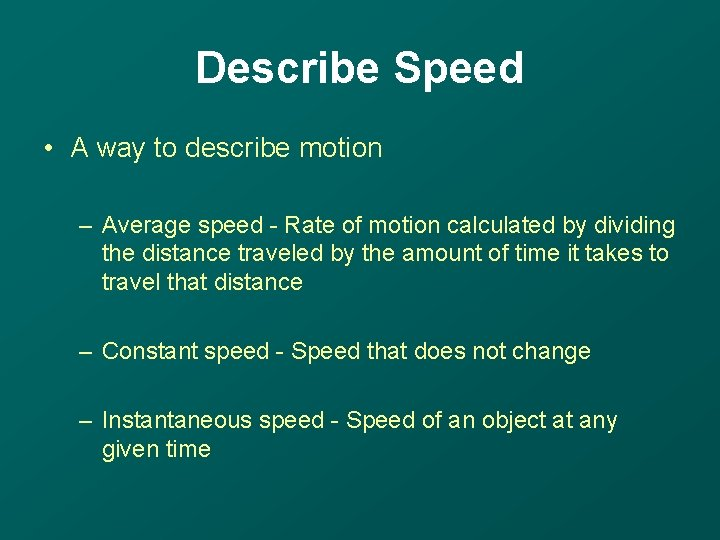Describe Speed • A way to describe motion – Average speed - Rate of motion calculated by dividing the distance traveled by the amount of time it takes to travel that distance – Constant speed - Speed that does not change – Instantaneous speed - Speed of an object at any given timeWhat is the formula used to calculate speed?What is the formula for calculating speed? Speed is calculated by dividing distance by time –Calculate This Speed A football field is about 100 m long. If it takes a person 20 seconds to run its length, how fast was the football player running?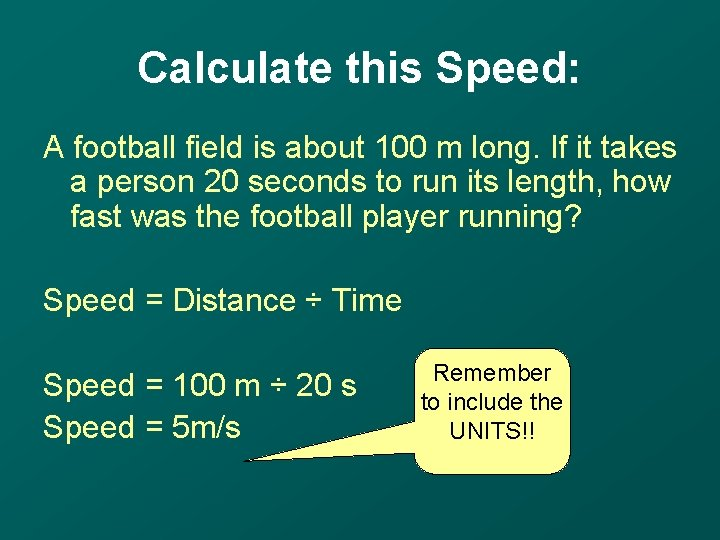Calculate this Speed: A football field is about 100 m long. If it takes a person 20 seconds to run its length, how fast was the football player running? Speed = Distance ÷ Time Speed = 100 m ÷ 20 s Speed = 5 m/s Remember to include the UNITS!!Explain Balanced Forces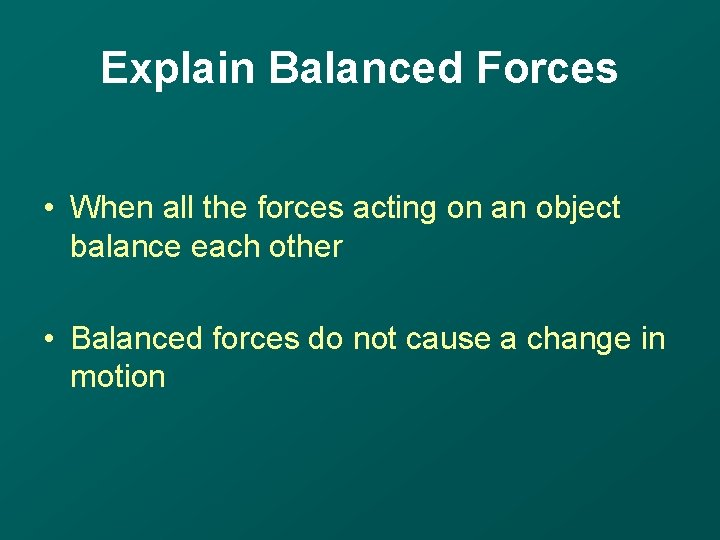Explain Balanced Forces • When all the forces acting on an object balance each other • Balanced forces do not cause a change in motionDescribe Friction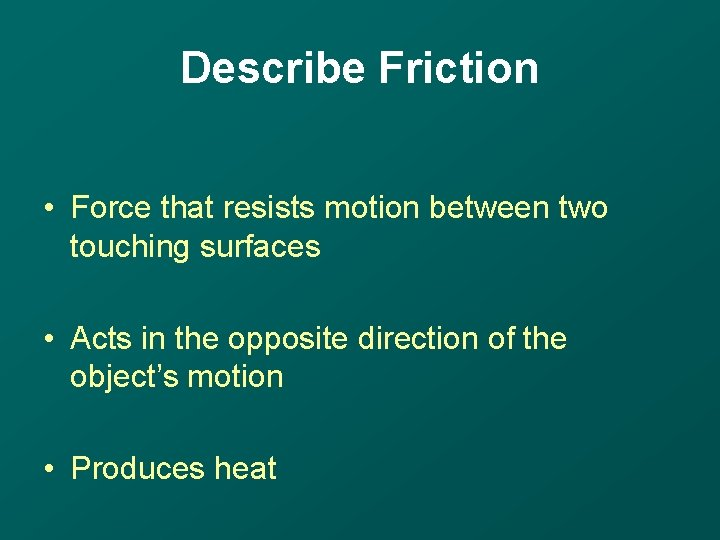Describe Friction • Force that resists motion between two touching surfaces • Acts in the opposite direction of the object’s motion • Produces heat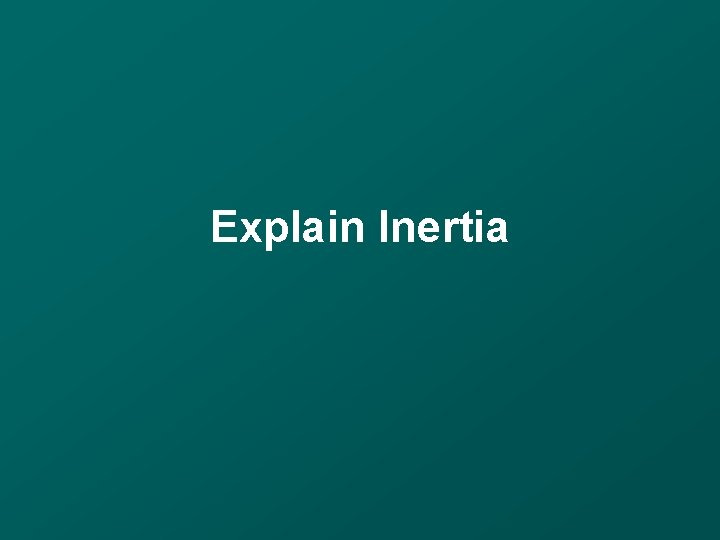Explain Inertia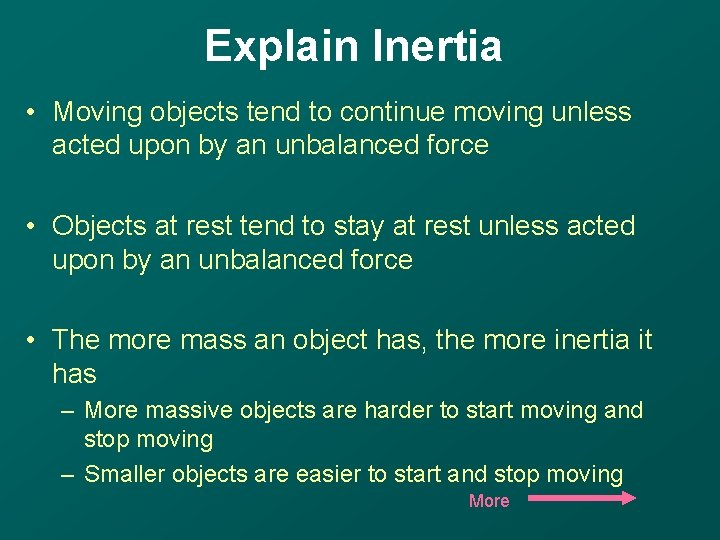Explain Inertia • Moving objects tend to continue moving unless acted upon by an unbalanced force • Objects at rest tend to stay at rest unless acted upon by an unbalanced force • The more mass an object has, the more inertia it has – More massive objects are harder to start moving and stop moving – Smaller objects are easier to start and stop moving MoreExplain Inertia Newton’s First Law on Motion describes the idea of inertia • An object at rest or in constant motion is acted upon by balanced forces – an unbalanced force will change the motion • Acceleration of an object at rest or in constant motion is 0 m/s/s (no motion)Explain Newton’s First Law of MotionExplain Newton’s First Law of Motion • Describes the idea of inertia Click the link below to observe the law http: //archive. ncsa. uiuc. edu/Cyberia/Video. Testbed/Projects/New. Physic s/newtons_1. html When you are finished, click the back button on your browser to return to this tutorial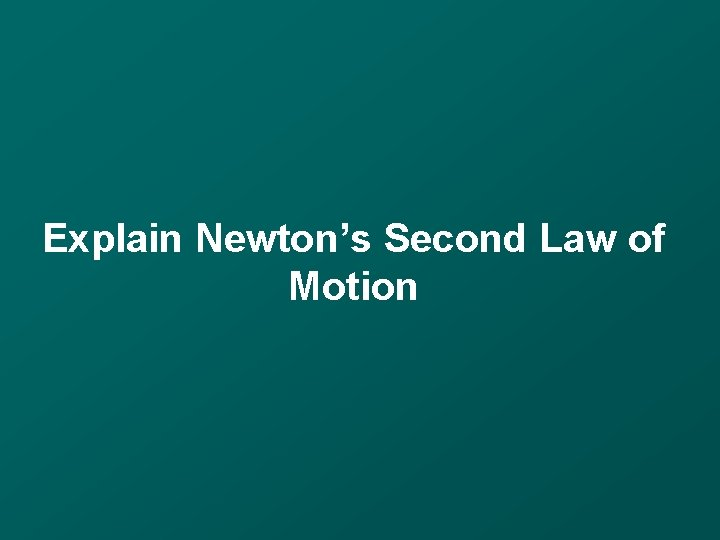Explain Newton’s Second Law of Motion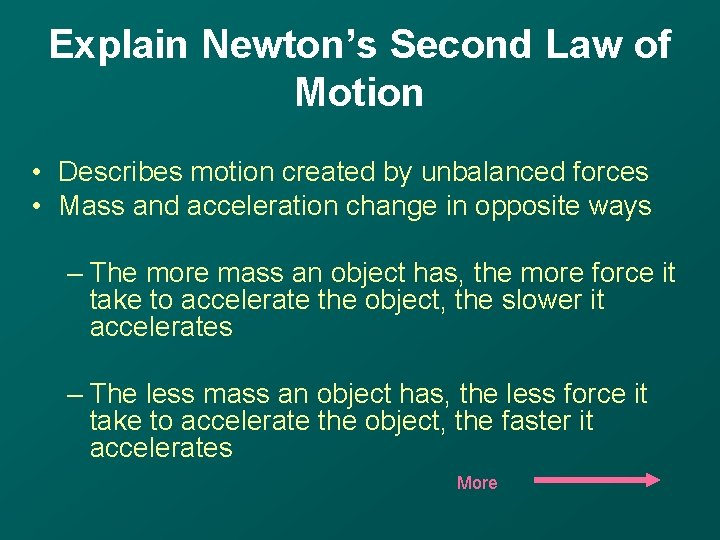Explain Newton’s Second Law of Motion • Describes motion created by unbalanced forces • Mass and acceleration change in opposite ways – The more mass an object has, the more force it take to accelerate the object, the slower it accelerates – The less mass an object has, the less force it take to accelerate the object, the faster it accelerates MoreExplain Newton’s Second Law of Motion Click on the link below to observe the law: http: //archive. ncsa. uiuc. edu/Cyberia/Video. Testbed/Projects/New. Physics/n ewtons_2. html When you are finished, click the back button on your browser to return to this tutorialExplain Newton’s Third Law of Motion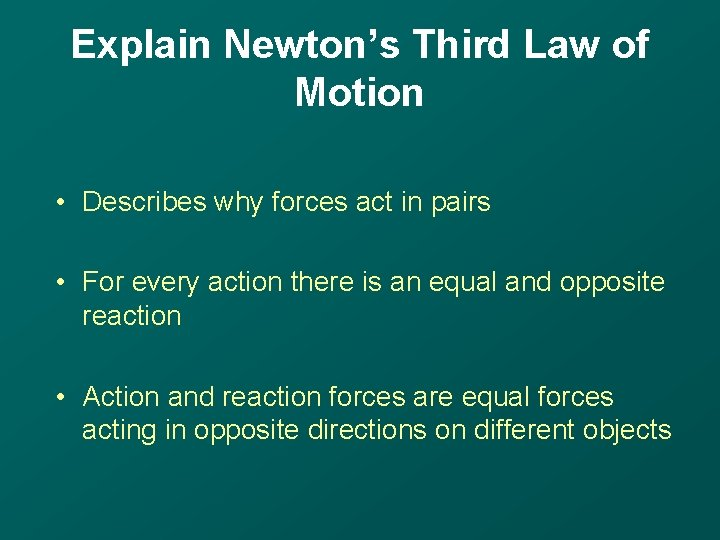Explain Newton’s Third Law of Motion • Describes why forces act in pairs • For every action there is an equal and opposite reaction • Action and reaction forces are equal forces acting in opposite directions on different objects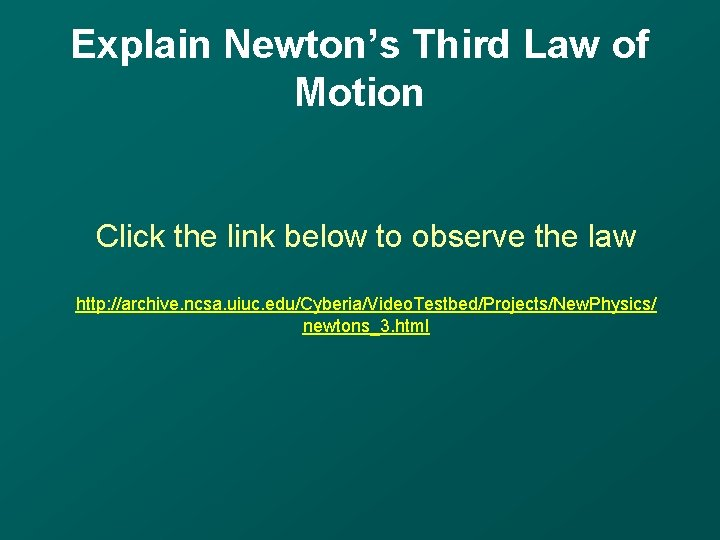Explain Newton’s Third Law of Motion Click the link below to observe the law http: //archive. ncsa. uiuc. edu/Cyberia/Video. Testbed/Projects/New. Physics/ newtons_3. htmlDistinguish Between Speed & Velocity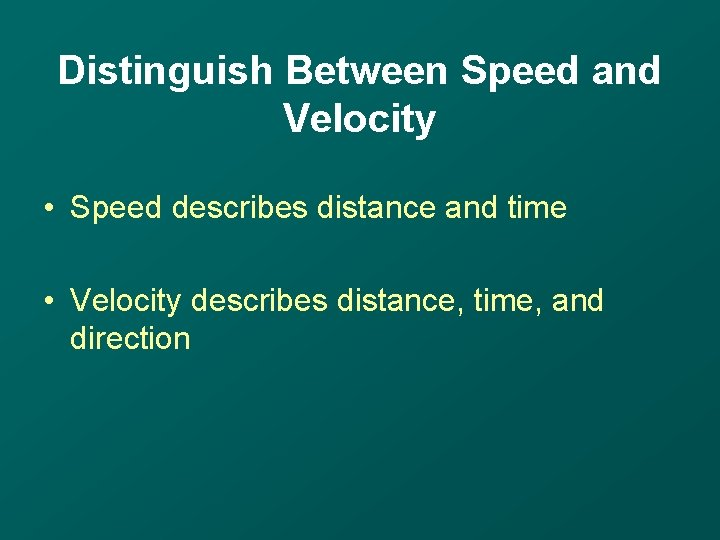Distinguish Between Speed and Velocity • Speed describes distance and time • Velocity describes distance, time, and direction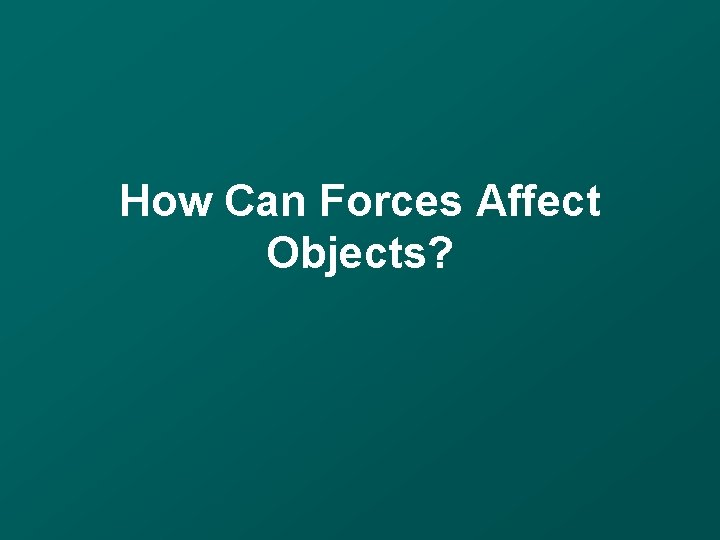How Can Forces Affect Objects?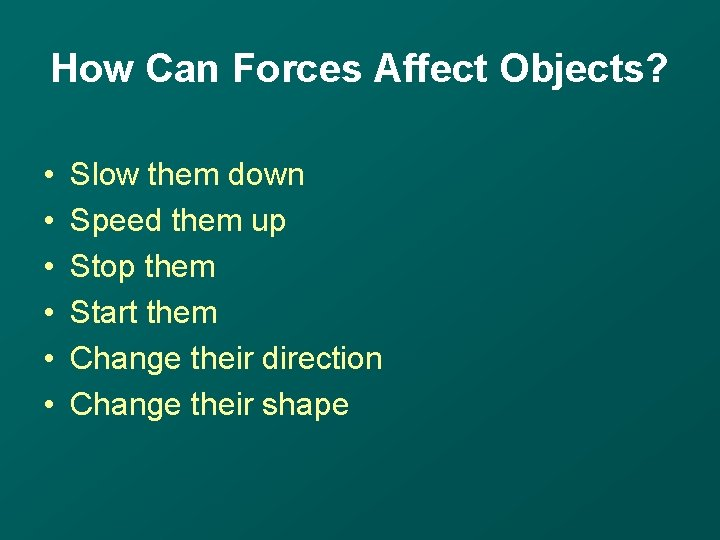How Can Forces Affect Objects? • • • Slow them down Speed them up Stop them Start them Change their direction Change their shapeInterpret The Graph Below: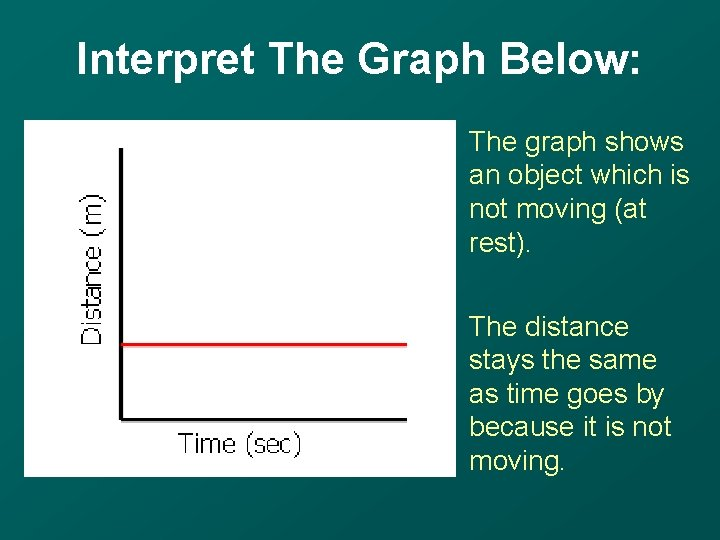Interpret The Graph Below: The graph shows an object which is not moving (at rest). The distance stays the same as time goes by because it is not moving.Interpret The Graph Below:Interpret The Graph Below: The graph shows that the objects distance increases as time passes. The object is moving and so it has velocity. The straight line shows it is a constant (not changing).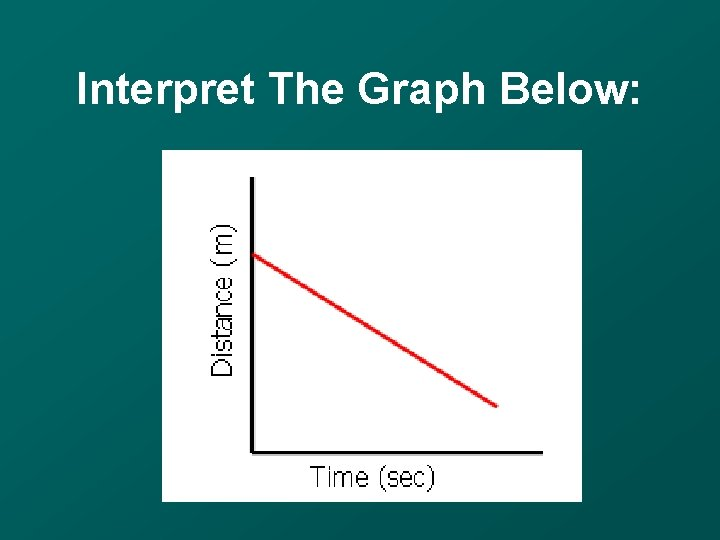Interpret The Graph Below: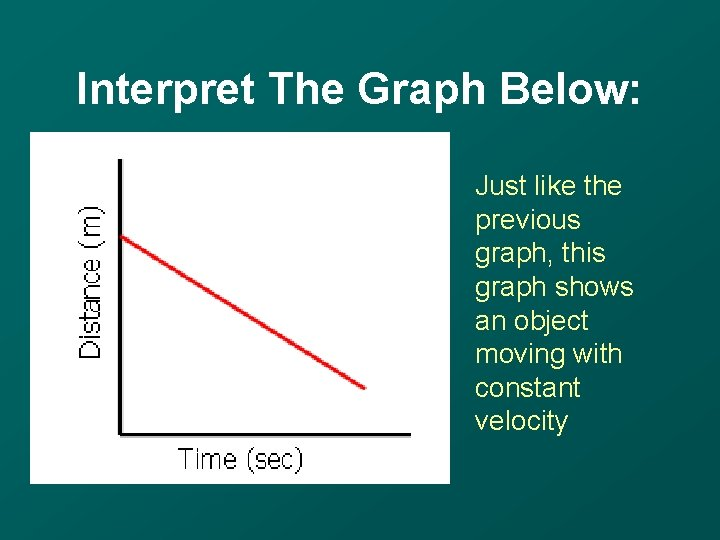Interpret The Graph Below: Just like the previous graph, this graph shows an object moving with constant velocity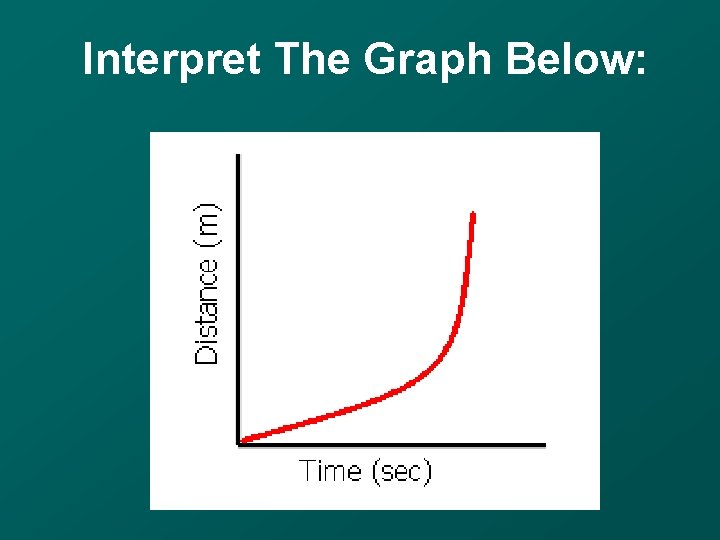Interpret The Graph Below:Interpret The Graph Below: The curve in the graph shows that the objects velocity is changing as time passes. This is acceleration.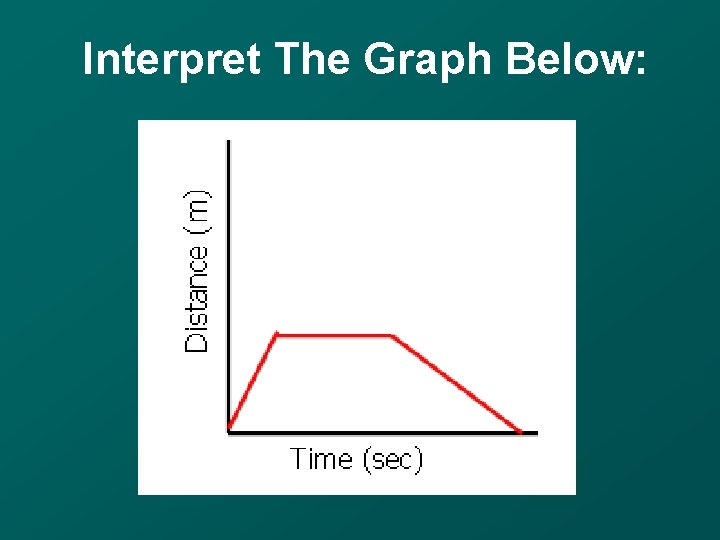Interpret The Graph Below: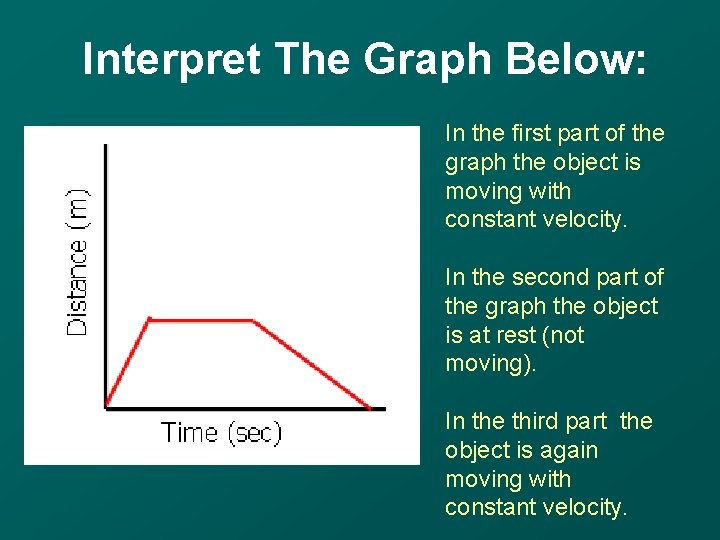Interpret The Graph Below: In the first part of the graph the object is moving with constant velocity. In the second part of the graph the object is at rest (not moving). In the third part the object is again moving with constant velocity.Interpret The Graph Below:Interpret The Graph Below: The graph shows that the objects velocity does not change as time passes. It shows constant velocity.Interpret The Graph Below: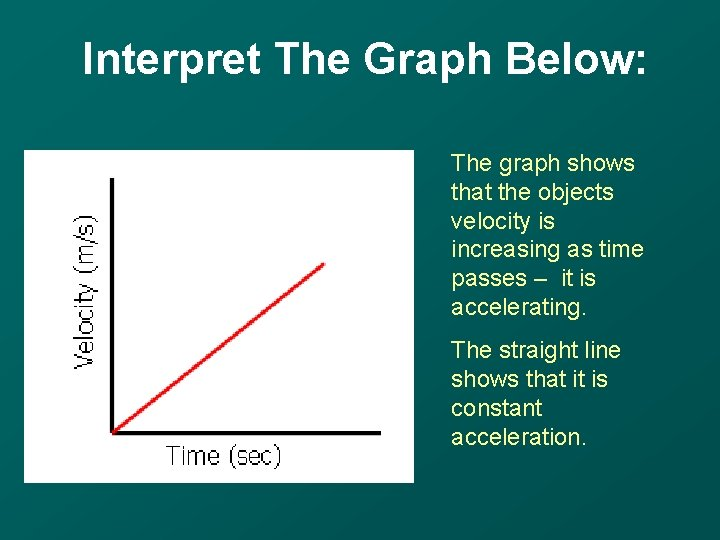Interpret The Graph Below: The graph shows that the objects velocity is increasing as time passes – it is accelerating. The straight line shows that it is constant acceleration.Click here for more review: http: //www. usoe. k 12. ut. us/curr/sci ence/sciber 00/8 th/forces/sciber/int ro. htm